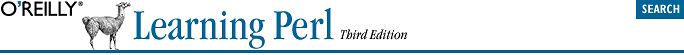home | O'Reilly's CD bookshelfs | FreeBSD | Linux | Cisco | Cisco Exam## 3.3. List Literals

```(1, 2, 3)      # list of three values 1, 2, and 3
(1, 2, 3,)     # the same three values (the trailing comma is ignored)
("fred", 4.5)  # two values, "fred" and 4.5
( )             # empty list - zero elements
(1..100)       # list of 100 integers```
```(1..5)            # same as (1, 2, 3, 4, 5)
(1.7..5.7)        # same thing - both values are truncated
(5..1)            # empty list - .. only counts "uphill"
(0, 2..6, 10, 12) # same as (0, 2, 3, 4, 5, 6, 10, 12)
(\$a..\$b)          # range determined by current values of \$a and \$b
(0..\$#rocks)      # the indices of the rocks array from the previous section```

As you can see from those last two items, the elements of an array are not necessarily constants -- they can be expressions that will be newly evaluated each time the literal is used. For example:

```(\$a, 17)       # two values: the current value of \$a, and 17
(\$b+\$c, \$d+\$e) # two values```

Of course, a list may have any scalar values, like this typical list of strings:

`("fred", "barney", "betty", "wilma", "dino")`